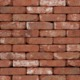•I didn't understand that calculation at all. I tried figuring it out and couldn't get it. Did you ever get an answer to that one?

•No, not great assistance on this one. Sorry Adrielle.

•Heyo. I can't recall, but I think they give you the following equation in the exam:

Delta(db) = 10 log (I/Io)

Essentially, the db change equals 10 log (ratio of intensities). In this case, the problem states that intensity was tripled (ie - 3/1).

Change in (db) = 10 log(3) = 4.77 db. 75 db + 4.77 db = 79.77 db (probably rounded to 80 on the exam).

Sorry for the formatting - does this make more sense?

•Anson, if the question asked for the raise in intensity, wouldn't the answer be just 4.77?